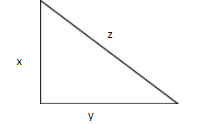: The perimeter of an isosceles triangle is 90 m. If the length of the two equal sides is $\dfrac{3}{4}$ of the length of the unequal side, find the dimensions of the triangle.Verified
146.4k+ views
Hint: With the given perimeter ,we can form a linear equation with two variables and by solving it we get the dimensions of the triangle.

Step 1:
It is given that it is an isosceles triangle. In an isosceles triangle any two sides are equal .
We are given the perimeter of the triangle and we know that the perimeter of the triangle is just the sum of its three sides.

Step 2 :Let the length of the sides be x , y , z.
Perimeter = x+y+z
Since it is an isosceles triangle, x = y
Therefore , perimeter = x + x +z
$\Rightarrow 90 = 2x + z$………….(1)
Step 3:
It is given that the length of the two equal sides is $\dfrac{3}{4}$ of the length of the unequal side.
$\Rightarrow x = \dfrac{3}{4}z$
Cross multiplying we get
$\Rightarrow 4x = 3z \\ \Rightarrow \dfrac{4}{3}x = z \\$
Now lets substitute the above value in equation (1)
$\Rightarrow 90 = 2x + \dfrac{4}{3}x$
Taking LCM , we get
$\Rightarrow 90 = \dfrac{{6x + 4x}}{3}$
Cross multiplying we get,
$\Rightarrow 270 = 10x \\ \Rightarrow \dfrac{{270}}{{10}} = x \\ \Rightarrow x = 27m \\$
Now we have obtained the value of x and as it is an isosceles triangle y = 27 m
Step 4 :
To find the value of z , substitute the value of x in equation (1)
$\Rightarrow 90 = 2(27) + z \\ \Rightarrow 90 = 54 + z \\ \Rightarrow 90 - 54 = z \\ \Rightarrow z = 36m \\$
Therefore the value of z=36m

Therefore, the dimensions of the isosceles triangle are 27 m , 27 m , 36 m.

Note: In this problem we have used a substitution method to solve the linear equation but we can also use elimination method to solve them.